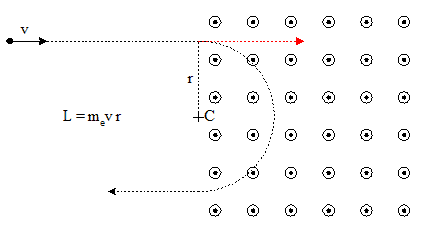# Classical EM vs Orbital Angular Momentum

Gold Member
Consider the following experiment from the point-of-view of classical mechanics and classical electromagnetism: An originally free electron then passes through a magnetic field that is oriented so that it causes the electron to turn to, say, the right. During the “turning” of the electron (a finite time interval), does it temporarily possesses classical orbital angular momentum?

Mentor
I'm not sure if there's any technical distinction between regular "angular momentum" and "orbital angular momentum", save that one is associated with an orbiting body.

Before the electron even enters the region of the magnetic field it already possesses angular momentum about the future center of the circular arc.•Dr_Nate, LarryS and vanhees71
During the “turning” of the electron (a finite time interval), does it temporarily possesses classical orbital angular momentum?
As @gneill notes: It has angular momentum even when it's moving in a straight line, around any point not on that line.

What exactly do you mean by "classical orbital angular momentum"? There is some abiguity:
https://en.wikipedia.org/wiki/Orbital_angular_momentum

Mentor
During the “turning” of the electron (a finite time interval), does it temporarily possesses classical orbital angular momentum?
Yes, except that it is probably much less temporary than you might assume.

QLogic
Edit: Ignore referframe. I thought you meant a quantum electron

Before the electron even enters the region of the magnetic field it already possesses angular momentum about the future center of the circular arc
Not exactly, although the distinction is a pedantic one to a certain degree in such a situation. It is still the case that one might measure the electron in a basis not commuting with Angular Momentum. Only if we have an experimental set up which can measure angular momentum can we then say (assuming the wave function is reasonably "tight" about a classical path) that it has orbital angular momentum.

Last edited:
Mentor
Not exactly, although the distinction is a pedantic one to a certain degree in such a situation. It is still the case that one might measure the electron in a basis not commuting with Angular Momentum. Only if we have an experimental set up which can measure angular momentum can we then say (assuming the wave function is reasonably "tight" about a classical path) that it has orbital angular momentum.
Keep in mind that this problem is posed in a "Classical Physics" domain.

•QLogic
QLogic
Keep in mind that this problem is posed in a "Classical Physics" domain.
Well if the electron is treated as a classical object then it definitely has orbital angular momentum. I thought usage of "temporarily possesses classical orbital angular momentum" suggested the electron was being treated quantum mechanically.

Mentor
one might measure the electron in a basis not commuting with Angular Momentum
I guess you missed the word “classical”. He is talking about a classical point charge, not an actual electron.

•QLogic
Mentor
Well if the electron is treated as a classical object then it definitely has orbital angular momentum. I thought usage of "temporarily possesses classical orbital angular momentum" suggested the electron was being treated quantum mechanically.
No harm, no foul. I frequently misinterpret intended situations until I stand back and look at the context.

•Dale and QLogic
Gold Member
I'm not sure if there's any technical distinction between regular "angular momentum" and "orbital angular momentum", save that one is associated with an orbiting body.

Before the electron even enters the region of the magnetic field it already possesses angular momentum about the future center of the circular arc.

View attachment 256352
Thanks, I forgot just how general the definition of classical angular momentum was.